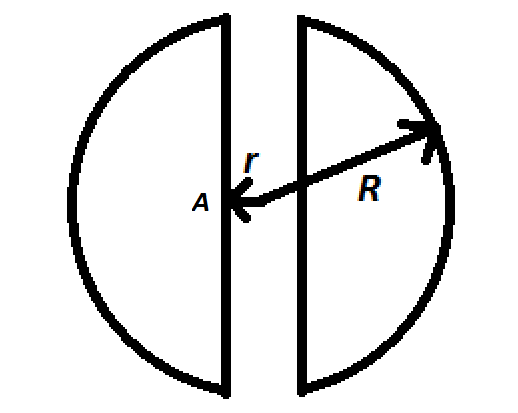# JEE Advanced Electrostatics (Non-Conductors) 2A cylindrical portion of radius $r$ is removed from a solid sphere of radius $R$ and uniform volume charge density $\rho$ in such a way that the axis of the hollow cylinder coincides with one of the diameters of the sphere. $(r<<. Then the electric field at point $A$ is

Assumptions

• $\hat{i}$ is a unit vector to the right
×